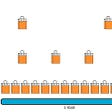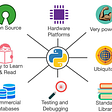# Pandas — Merging, Joining & Concatenations

## Facilities For Easily Combining Together Series or DataFrame — #PySeries#Episode 13

`print(“Hello Pandas — Merging Joining and Concatenating”)import numpy as npimport pandas as pd`

Preparing the 3 DataFrame:

`df1=pd.DataFrame({'A':['A0','A1','A2','A3'],'B':['B0','B1','B2','B3'],'C':['C0','C1','C2','C3'],'D':['D0','D1','D2','D3']},index=[0,1,2,3])df2=pd.DataFrame({'A':['A4','A5','A6','A7'],'B':['B4','B5','B6','B7'],'C':['C4','C5','C6','C7'],'D':['D4','D5','D6','D7']},index=[0,1,2,3])df3=pd.DataFrame({'A':['A8','A9','A10','A11'],'B':['B8','B9','B10','B11'],'C':['C8','C9','C10','C11'],'D':['D8','D9','D10','D11']},index=[0,1,2,3])`

## Concatenation

Basically glues together DataFrames; keep in mind that dimensions should match along the axis we are concatenation on. We can use pd.concat() and pass in a list of DataFrames to concatenation together.

Combine two DataFrame objects with identical columns:

`pd.concat([df1,df2,df3])`
`pd.concat([df1,df2,df3], axis=1)`

## Merging: Single key

Merging allows us to merge DataFrames together using similar logic as mapping SQL.

2 More DataFrame:

`left=pd.DataFrame({'key':['K0','K1','K2','K3'],'A':['A0','A1','A2','A3'],'B':['B0','B1','B2','B3'],})left`
`right=pd.DataFrame({'key':['K0','K1','K2','K3'],'A':['A4','A5','A6','A7'],'B':['B4','B5','B6','B7'],})right`
`pd.merge(left,right,how='inner',on='key')`

## Merging: Multiply keys

DataFrames are now merged into a single DataFrame based on the common values present in the id column of both the DataFrames

`left = pd.DataFrame({'key1': ['K0', 'K0', 'K1', 'K2'],'key2': ['K0', 'K1', 'K0', 'K1'],'A': ['A0', 'A1', 'A2', 'A3'],'B': ['B0', 'B1', 'B2', 'B3']})left`
`right = pd.DataFrame({'key1': ['K0', 'K1', 'K1', 'K2'],'key2': ['K0', 'K0', 'K0', 'K0'],'C': ['C0', 'C1', 'C2', 'C3'],'D': ['D0', 'D1', 'D2', 'D3']})right`

## Merging on Multiply keys

`pd.merge(left,right,on=['key1','key2'])`
`pd.merge(left,right,how='outer',on=['key1','key2'])`
`pd.merge(left,right,how=’right’,on=[‘key1’,’key2'])`
`pd.merge(left,right,how='left',on=['key1','key2'])`

## Joining

Joining is a convenient method for combining the two columns of the two potentially differently-indexed DataFrame into a single result DataFrame;

Same as Merging, except the *key* is an index, instead of columns

`left = pd.DataFrame({'A': ['A0', 'A1', 'A2'],'B': ['B0', 'B1', 'B2']},index=['K0', 'K1', 'K2'])right = pd.DataFrame({'C': ['C0', 'C2', 'C3'],'D': ['D0', 'D2', 'D3']},index=['K0', 'K2', 'K3'])left`
`right`
`left.join(right)`
`left.join(right, how='outer')`

# Credits & References:

Jose Portilla — Python for Data Science and Machine Learning Bootcamp — Learn how to use NumPy, Pandas, Seaborn , Matplotlib , Plotly , Scikit-Learn , Machine Learning, Tensorflow , and more!

# Posts Related:

00Episode#PySeries — Python — Jupiter Notebook Quick Start with VSCode — How to Set your Win10 Environment to use Jupiter Notebook

01Episode#PySeries — Python — Python 4 Engineers — Exercises! An overview of the Opportunities Offered by Python in Engineering!

02Episode#PySeries — Python — Geogebra Plus Linear Programming- We’ll Create a Geogebra program to help us with our linear programming

03Episode#PySeries — Python — Python 4 Engineers — More Exercises! — Another Round to Make Sure that Python is Really Amazing!

04Episode#PySeries — Python — Linear Regressions — The Basics — How to Understand Linear Regression Once and For All!

05Episode#PySeries — Python — NumPy Init & Python Review — A Crash Python Review & Initialization at NumPy lib.

06Episode#PySeries — Python — NumPy Arrays & Jupyter Notebook — Arithmetic Operations, Indexing & Slicing, and Conditional Selection w/ np arrays.

07Episode#PySeries — Python — Pandas — Intro & Series — What it is? How to use it?

08Episode#PySeries — Python — Pandas DataFrames — The primary Pandas data structure! It is a dict-like container for Series objects

09Episode#PySeries — Python — Python 4 Engineers — Even More Exercises! — More Practicing Coding Questions in Python!

10Episode#PySeries — Python — Pandas — Hierarchical Index & Cross-section — Open your Colab notebook and here are the follow-up exercises!

11Episode#PySeries — Python — Pandas — Missing Data — Let’s Continue the Python Exercises — Filling & Dropping Missing Data

12Episode#PySeries — Python — Pandas — Group By — Grouping large amounts of data and compute operations on these groups

13Episode#PySeries — Python —Pandas — Merging, Joining & Concatenations — Facilities For Easily Combining Together Series or DataFrame (this one)

14Episode#PySeries — Python — Pandas — Pandas Dataframe Examples: Column Operations

15Episode#PySeries — Python — Python 4 Engineers — Keeping It In The Short-Term Memory — Test Yourself! Coding in Python, Again!

16Episode#PySeries — NumPy — NumPy Review, Again;) — Python Review Free Exercises

17Episode#PySeriesGenerators in Python — Python Review Free Hints

18Episode#PySeries — Pandas Review…Again;) — Python Review Free Exercise

19Episode#PySeriesMatlibPlot & Seaborn Python Libs — Reviewing theses Plotting & Statistics Packs

20Episode#PySeriesSeaborn Python Review — Reviewing theses Plotting & Statistics Packs

--

--

--

## More from Jungletronics

J of Jungle + 3 Plats Arduino/RPi/Pic = J3

## Collaborative Filtering based Recommendation Systems exemplified..## This will make you get by with Airbnb in Boston## Use This API To Create Charts For Crude Oil Prices## Your metrics may be more arbitrary (and less useful) than you think.## Udacity DataVisualization Nanodegree Capstone Project## How to Create an Interactive Startup Growth Calculator with Python## J3

Hi, Guys o/ I am J3! I am just a hobby-dev, playing around with Python, Django, Lego, Arduino, Raspy, PIC, AI… Welcome! Join us!

## Real Python